# Community

Blog Events Webinars Tutorials Forum
×
Community Blog Use Mars with RAPIDS to Accelerate Data Science on GPUs in Parallel Mode

# Use Mars with RAPIDS to Accelerate Data Science on GPUs in Parallel Mode

The author explains the commonalities and differences of code within common Python tools, Mars, and RAPIDS, and how it can pave the way for the future of data science.

By Ji Sheng

## Background

In the data science field, Python is becoming increasingly crucial. The tools used in Python include NumPy, pandas, and scikit-learn.

### NumPy

NumPy is the fundamental package for computing. It provides the N-dimensional array (ndarray) data structure to facilitate computing in any dimension.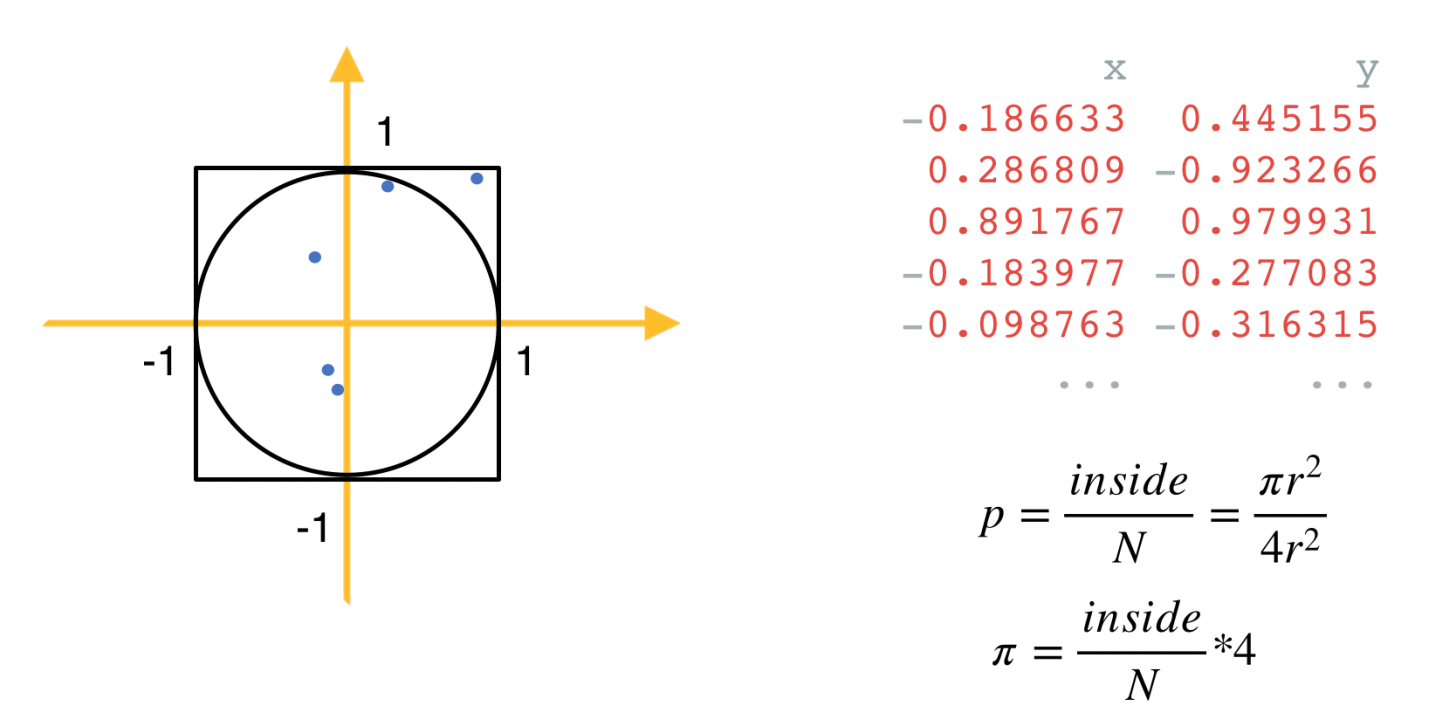Let's use the Monte Carlo method to calculate pi. The principle is simple. As shown in the preceding figure, we have a circle with a radius of 1 and a square with a side length of 2, both centered on the original point. We can generate many points evenly distributed in the square and then calculate the value of pi by using the formula: Pi = Number of points in the circle/Total number of points x 4.

The more randomly generated points we have, the more accurate the result.

NumPy is used for computing:

``````import numpy as np

N = 10 ** 7  # 1千万个点

data = np.random.uniform(-1, 1, size=(N, 2))  # 生成1千万个x轴和y轴都介于-1和1间的点
inside = (np.sqrt((data ** 2).sum(axis=1)) < 1).sum()  # 计算到原点的距离小于1的点的个数
pi = 4 * inside / N
print('pi: %.5f' % pi)``````

As you can see, NumPy can implement computing by using only a few lines of code. After you get used to the array-oriented NumPy, you can use it to greatly improve code readability and execution efficiency.

### Pandas

Pandas is a powerful tool for data analysis and processing. It provides a massive number of APIs that can be used to analyze and process data in DataFrame.

DataFrame is a core data structure of pandas. It can be simply understood as table data, but it contains row- and column-based indexes. However, such indexes are different from those in databases. We can think of DataFrame as a dictionary of row-based indexes and data or a dictionary of column-based indexes and data. You can easily select a row or a column of data based on the row-based or column-based indexes.

Let's use a MovieLens dataset, specifically the MovieLens 20M Dataset, to see how pandas is used.

``````import pandas as pd

ratings.groupby('userId').agg({'rating': ['sum', 'mean', 'max', 'min']})``````

According to `pandas.read_csv`, we can read CSV data, group and aggregate data by user ID, and calculate the sum, average, maximum, and minimum values of the rating column in each group.

The best way to use pandas is to analyze data interactively in Jupyter Notebook.

### Scikit-learn

Scikit-learn is a library used for machine learning in Python. You can complete machine learning tasks through several simple high-level APIs without having to pay attention to algorithm details. Although deep learning is used in many algorithms, scikit-learn can still be used as a basic machine learning library to streamline the entire process.

Let's use K-Nearest Neighbor (KNN) as an example to see how we can use scikit-learn to complete a task.

``````import pandas as pd
from sklearn.neighbors import NearestNeighbors

df = pd.read_csv('data.csv')  # 输入是 CSV 文件，包含 20万个向量，每个向量10个元素
nn = NearestNeighbors(n_neighbors=10)
nn.fit(df)
neighbors = nn.kneighbors(df)``````

`fit` is the most common API for learning in scikit-learn. As you can see, the whole process is very simple and easy to understand.

### Mars: Parallel and Distributed Accelerator for NumPy, Pandas, and Scikit-learn

The data science stack of Python is powerful but it has the following problems:

1. Multi-core capabilities can be used for new operations in these libraries.
2. As deep learning becomes more popular, more and more new hardware for accelerating data science is emerging. The most common hardware is the graphics processing unit (GPU) but can we use GPUs to accelerate data processing in the preorder process of deep learning?
3. The operations of these libraries are all imperative, not declarative. Focusing on telling the system how to do something, imperative operations can obtain results immediately, facilitating result exploring. Imperative operations are flexible but occupy a lot of memory resources that cannot be released quickly. Imperative operations are separated from each other. Operator fusion cannot be performed to improve performance. In contrast, declarative operations focus on telling the system what to do and are more concerned with the results. Typical declarative operations such as SQL statements and TensorFlow 1.x operations can be performed only when the results are truly needed. This is a lazy evaluation. During the process, a lot of optimization can be performed to improve performance. However, declarative operations are not flexible and are hard to debug.

To solve these problems, Mars was developed by the MaxCompute team. It aims to enable data science libraries such as NumPy, pandas, and scikit-learn to be executed in a parallel and distributed manner, so that the multi-core capabilities and new hardware can be fully utilized.

During the development of Mars, we focused on the following features:

1. We wanted Mars to be simple enough so that anyone who knows NumPy, pandas, or scikit-learn can use Mars.
2. We wanted the functions or features of these libraries to be reusable after the libraries are scheduled to multiple cores or multiple workers.
3. We wanted to allow users to switch between declarative operations and imperative operations to achieve both flexibility and performance.
4. Mars should be robust enough for production and able to cope with various failovers.

These were our goals and direction of our efforts.

### Mars Tensor: Parallel and Distributed Accelerator for NumPy

As mentioned above, we wanted to make it easy for anyone who knows NumPy, pandas, or scikit-learn to use Mars. Let's use Monte Carlo as an example:

``````import mars.tensor as mt

N = 10 ** 10

data = mt.random.uniform(-1, 1, size=(N, 2))
inside = (mt.sqrt((data ** 2).sum(axis=1)) < 1).sum()
pi = (4 * inside / N).execute()
print('pi: %.5f' % pi)``````

As you can see, `import numpy as np` is changed to `import mars.tensor as mt`. All subsequent instances of `np.` are changed to `mt.` and the `.execute()` method is called before pi is printed.

That means, by default, Mars migrates code in a declarative manner with very low costs. You can use the `.execute()` method to get data when needed. This can maximize performance and reduce memory consumption.

Here, we have also expanded the data scale by a factor of 1,000 to 10 billion points. It took 757 milliseconds to process 10 million points (1/1000) on my notebook. Now, the data volume increases by a factor of 1,000 and 150 GB memory is required for processing `data`. The whole task cannot be completed by NumPy alone. In contrast, Mars spends only 3 minutes and 44 seconds on computing, with a peak memory usage of only 1 GB. Assuming that the memory size is infinitely large, the time required by NumPy is more than 12 minutes, an increase by a factor of 1,000. In contrast, Mars can make full use of multi-core capabilities and use declarative operations to greatly reduce the memory usage.

As mentioned above, we have tried to use both the declarative and imperative styles. To use the imperative style, we only need to configure an option at the beginning of the code.

``````import mars.tensor as mt
from mars.config import options

options.eager_mode = True  # 打开 eager mode 后，每一次调用都会立即执行，行为和 Numpy 就完全一致

N = 10 ** 7

data = mt.random.uniform(-1, 1, size=(N, 2))
inside = (mt.linalg.norm(data, axis=1) < 1).sum()
pi = 4 * inside / N  # 不需要调用 .execute() 了
print('pi: %.5f' % pi.fetch())  # 目前需要 fetch() 来转成 float 类型，后续我们会加入自动转换``````

### Mars DataFrame: Parallel and Distributed Accelerator for pandas

Migrating code from pandas to Mars DataFrame is similar to migrating code from NumPy to Mars tensor, with only two differences. Let's use the MovieLens code as an example:

``````import mars.dataframe as md

ratings.groupby('userId').agg({'rating': ['sum', 'mean', 'max', 'min']}).execute()``````

### Mars Learn: Parallel and Distributed Accelerator for Scikit-learn

Migrating code from scikit-learn to Mars Learn is also similar. Mars Learn only supports a few scikit-learn algorithms but we are working hard to migrate the algorithm code to it. Anyone interested is welcome to join us.

``````import mars.dataframe as md
from mars.learn.neighbors import NearestNeighbors

df = md.read_csv('data.csv')  # 输入是 CSV 文件，包含 20万个向量，每个向量10个元素
nn = NearestNeighbors(n_neighbors=10)
nn.fit(df)  # 这里 fit 的时候也会整体触发执行，因此机器学习的高层接口都是立即执行的
neighbors = nn.kneighbors(df).fetch()  # kneighbors 也已经触发执行，只需要 fetch 数据``````

Note that Mars Learn can immediately run the `fit` and `predict` APIs for machine learning to ensure the semantic correctness.

## RAPIDS: Data Science on GPUs

You may have noticed that, so far, we have not mentioned GPUs. Now, let's talk about RAPIDS, a GPU-accelerated data science platform.

Although Compute Unified Device Architecture (CUDA) has greatly reduced the difficulty of GPU programming, it is almost impossible for data scientists to use a GPU to process the data that NumPy and pandas can process. Fortunately, NVIDIA provides an open-source RAPIDS platform. Similar to Mars, RAPIDS allows you to migrate code from NumPy, pandas, and scikit-learn to a GPU through import statements.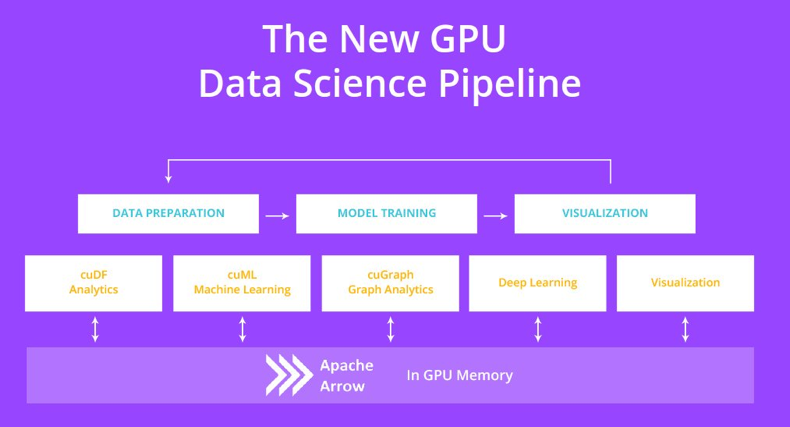RAPIDS cuDF is used to accelerate pandas, while RAPIDS cuML is used to accelerate scikit-learn.

For NumPy, CuPy can be accelerated by using GPUs, allowing RAPIDS to focus on other parts of data science.

### CuPy: GPU-based Accelerator of NumPy

Let's use Monte Carlo as an example to calculate pi:

``````import cupy as cp

N = 10 ** 7

data = cp.random.uniform(-1, 1, size=(N, 2))
inside = (cp.sqrt((data ** 2).sum(axis=1)) < 1).sum()
pi = 4 * inside / N
print('pi: %.5f' % pi)``````

In my test, CuPy reduced the CPU time consumption by more than 2000%. It dropped from 757 milliseconds to 36 milliseconds since a GPU is suitable for computing-intensive tasks.

### RAPIDS cuDF: GPU-based Accelerator of Pandas

The code `import pandas as pd` is changed to `import cudf`. You do not need to be concerned with the parallel implementation in the GPU or CUDA programming.

``````import cudf

ratings.groupby('userId').agg({'rating': ['sum', 'mean', 'max', 'min']})``````

The runtime is reduced by over 1000%, from 18s on the CPU to 1.66s on a GPU.

### RAPIDS cuML: GPU-based Accelerator of Scikit-learn

Let's continue using KNN as an example:

``````import cudf
from cuml.neighbors import NearestNeighbors

nn = NearestNeighbors(n_neighbors=10)
nn.fit(df)
neighbors = nn.kneighbors(df)``````

The runtime is reduced from 1 minute and 52 seconds on a CPU to 17.8 seconds on a GPU.

## Benefits of Using Mars with RAPIDS

RAPIDS implements Python data science on a GPU, greatly improving the runtime efficiency for data science operations. They also use the imperative style. When Mars and RAPIDS are used together, less memory is consumed in the process, allowing more data to be processed. Mars can also distribute computing to multiple workers and GPUs to improve the data scale and computing efficiency.

It is easy to use a GPU in Mars. You only need to specify `gpu=True` for corresponding functions. For example, a GPU can be used to create tensors and read CSV files.

``````import mars.tensor as mt
import mars.dataframe as md

a = mt.random.uniform(-1, 1, size=(1000, 1000), gpu=True)

The following figure shows how Mars accelerates pi calculation in scale-up and scale-out dimensions by using Monte Carlo methods. Generally, we can accelerate a data science task in either of two ways: Scale-up means using better hardware, such as a better CPU, a larger memory, or a GPU, while scale-out means using more workers to improve efficiency in a distributed manner.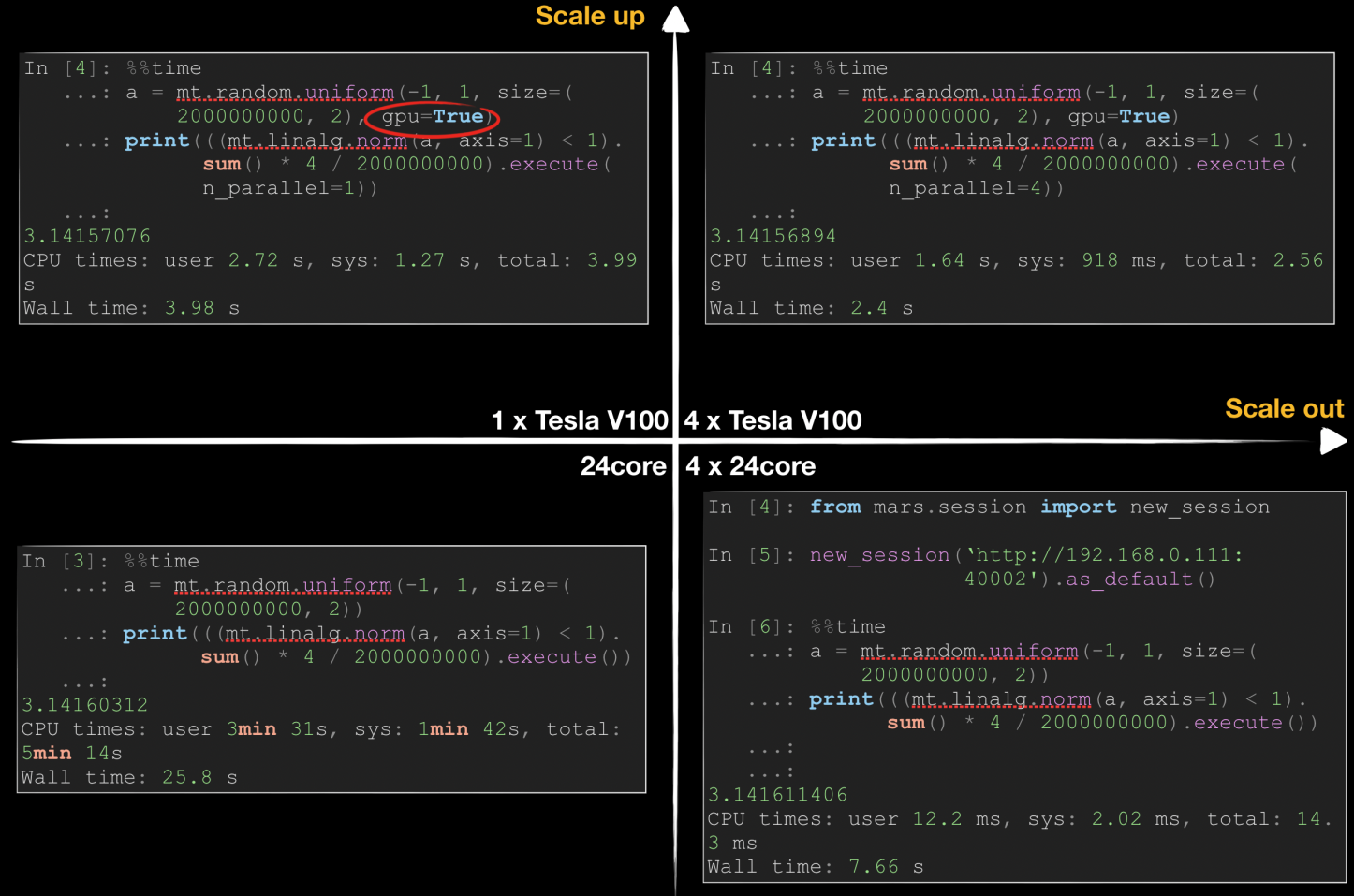As shown in the preceding figure, Mars requires 25.8 seconds for computing on one 24-core server, while the time is linearly reduced in distributed mode when four 24-core servers are used. By using NVIDIA Tesla V100, we can reduce the runtime on a single server to 3.98 seconds, which surpasses the performance of 4 CPUs. By using multiple GPUs, we can further reduce the runtime. It is difficult to linearly reduce the runtime because the network and data replication overhead increase significantly.

## Performance Testing

We used the dataset on Github to test three GROUP BY clauses and one join clause and compare pandas with DASK. Both DASK and Mars were built to implement Python data science operations in a parallel and distributed manner but are significantly different in design, implementation, and distribution.

The test server is configured with 500 GB memory, 96 cores, and an NVIDIA V100 GPU. Both Mars and DASK use RAPIDS to perform computing on GPUs.

### GROUP BY

The data sizes are 500 MB, 5 GB, and 20 GB.

There are also three groups of queries.

### Query 1

``````df = read_csv('data.csv')
df.groupby('id1').agg({'v1': 'sum'})``````

### Query 2

``````df = read_csv('data.csv')
df.groupby(['id1', 'id2']).agg({'v1': 'sum'})``````

### Query 3

``````df = read_csv('data.csv')
df.gropuby(['id6']).agg({'v1': 'sum', 'v2': 'sum', 'v3': 'sum'})``````

### Performance Testing Result for 500 MB Data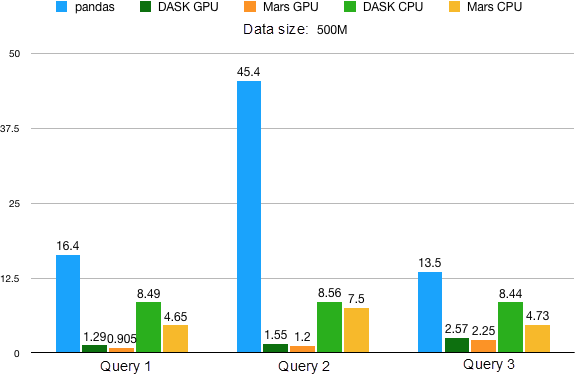### Performance Testing Result for 5 GB Data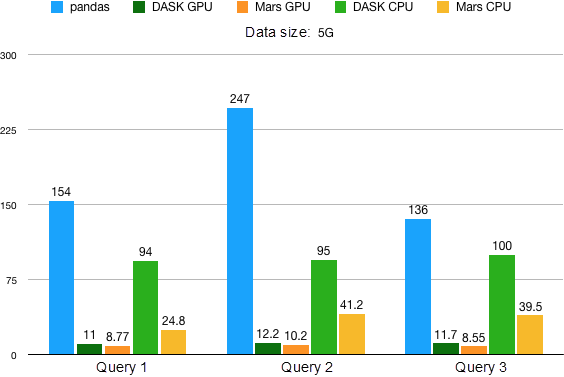### Performance Testing Result for 20 GB Data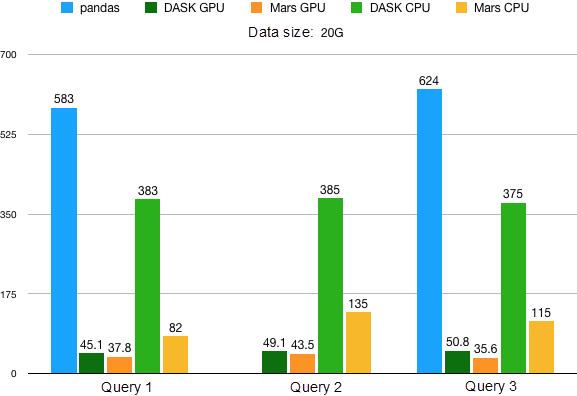When the data size reaches 20 GB, out of memory (OOM) occurs in pandas in query 2, and no results can be obtained.

The performance advantages of Mars are more obvious as the data size increases.

Due to the powerful computing capabilities of the GPU, the operation performance of the GPU is several times greater than that of the CPU. When only RAPIDS cuDF is used, the tests fail when the data size reaches 5 GB due to the limitation of the video memory size. When Mars is used, the use of video memory can be greatly optimized during declarative operations. All of the tests can be easily completed even if the data size reaches 20 GB. This is the power of Mars and RAPIDS when used together.

### Join

Test query:

``````x = read_csv('x.csv')
x.merge(y, on='id1')``````

In the test data, x indicates 500 MB while y indicates 10 lines of data.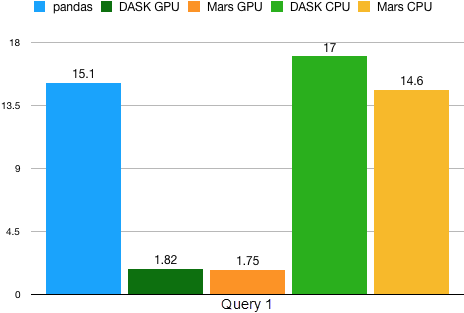## Summary

RAPIDS implements Python data science operations on a GPU, greatly improving the data analysis and processing efficiency. Mars focuses more on data processing in a parallel and distributed manner. Their combination opens up many areas of exploration for the future.

Mars was developed by the MaxCompute team. MaxCompute is an efficient and fully-managed data warehouse solution that can process exabytes of data. Mars will soon be available on MaxCompute, giving users an out-of-the-box experience of Mars services. This data migration plan will be soon available.

0 0 0
Share on

# Related Products

• ##Machine Learning Platform For AI

An end-to-end platform that provides various machine learning algorithms to meet your data mining and analysis requirements.

• ##Epidemic Prediction Solution

This technology can be used to predict the spread of COVID-19 and help decision makers evaluate the impact of various prevention and control measures on the development of the epidemic.

• ##Online Education Solution

This solution enables you to rapidly build cost-effective platforms to bring the best education to the world anytime and anywhere.

• ##Accelerated Global Networking Solution for Distance Learning

Alibaba Cloud offers an accelerated global networking solution that makes distance learning just the same as in-class teaching.